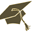Thesis (Selection of subject)(version: 290)
Assignment details
Login via CASTermodynamická interpretace Landauova tlumení
Thesis title in Czech: Termodynamická interpretace Landauova tlumení Thermodynamic interpretation of Landau damping Vlasovova rovnice, Landauovo tlumení, nerovnovážná termodynamika, entropie Vlasov equation, Landau damping, non-equilibrium thermodynamics, entropy 2018/2019 dissertation Mathematical Institute of Charles University (32-MUUK) RNDr. Michal Pavelka, Ph.D.
 Guidelines In thermodynamics, relaxation to equilibrium should be accompanied by growth of entropy (the second law of thermodynamics). Since Boltzmann entropy is conserved by Vlasov equation, Landau damping (relaxation to spatially homogeneous equilibria), seems to be incompatible with thermodynamics at first sight. The goal of this work is to regard Vlasov equation from the perspective of multiscale non-equilibrium thermodynamics and to study the possibility to find an entropy that grows during Landau damping. That could push forward the important problem of understanding Landau damping much further.
 References  C. Villani and C. Mohout, On Landau damping, Acta Mathematica 207 (1), 2011, pp 29-201
 Preliminary scope of work in English State of plasma (or a collection of particles with long range interactions) can be described by means of one-particle distribution function f(r,p), which depends on both space and momentum. Evolution of this distribution function is given by Vlasov equation (continuity equation in phase space), which is the Boltzmann equation without the collision integral, and where the potential energy is expressed in the mean field approximation. Vlasov equation is a reversible evolution equation in the sense that when inverting velocities of particles, the backward motion is solution of the same equation as the forward motion. This is also reflected in conservation of Boltzmann entropy by Vlasov equation. Although the equation is reversible, it exhibits remarkable behavior – relaxation to spatially homogenenous distribution functions. That behavior is referred to as Landau damping. The tendency towards spatially homogeneous distribution was originally discovered by L.D. Landau for the linearized Vlasov equation while the fully nonlinear case was proved by C. Mouhot and C. Villani in 2011 , for which the latter author received Fields medal. In thermodynamics, relaxation to equilibrium should be accompanied by growth of entropy (the second law of thermodynamics). Since Boltzmann entropy is conserved by Vlasov equation, Landau damping (relaxation to spatially homogeneous equilibria), seems to be incompatible with thermodynamics at first sight. The goal of this work is to regard Vlasov equation from the perspective of multiscale non-equilibrium thermodynamics and to study the possibility to find an entropy that grows during Landau damping. That could push forward the important problem of understanding Landau damping much further. The impact of such thermodynamic interpretation would be enormous. Firstly, it would elucidate the physical meaning Landau damping. Secondly, it would open the possibility of application to similar systems as Landau damping in collective motion of stars and galaxies. Finally, it would improve the state of the art understanding of non-equilibrium thermodynamic.

Charles University | Information system of Charles University | http://www.cuni.cz/UKEN-329.html